# The Most Common Digital SAT Math Topics: Part 1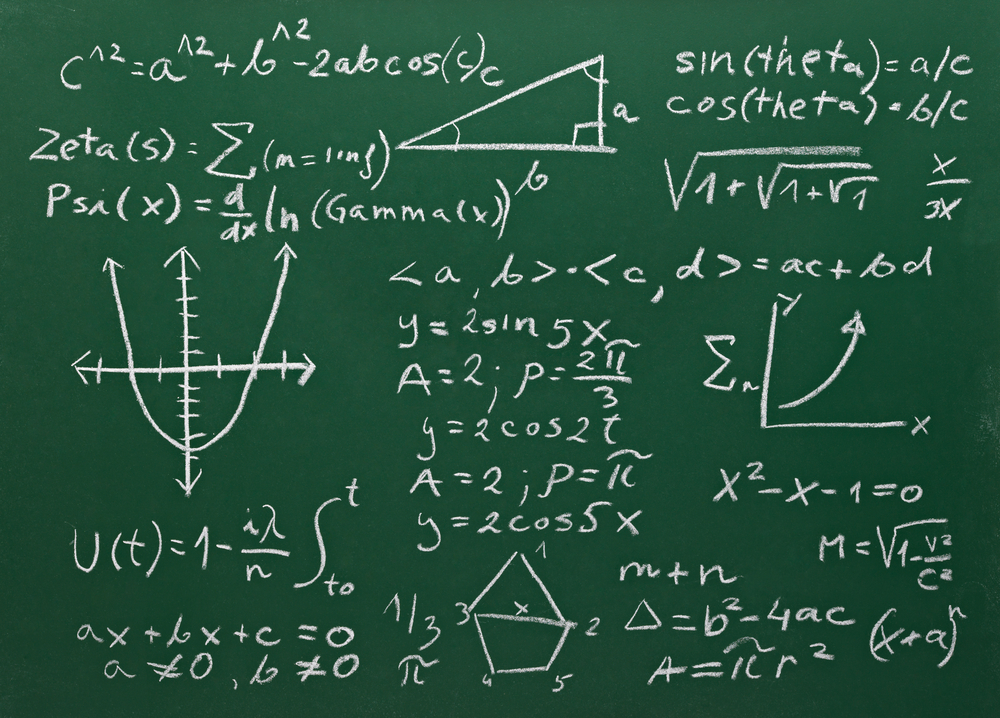The current SAT math section spans a wide range of topics, but if you look closely, you’ll notice that certain concepts and question types show up on almost every test. The digital SAT math section will be no different. The goal of this blog post isn’t to cover every topic that could appear on the digital SAT math section—you wouldn’t want to read something that long!—rather, it’s to show you that the section is predictable, its most frequently recurring topics relatively easy to manage.

In our deep dive into the four new digital SAT practice tests, we found that the math topics you’re most likely to see on the digital SAT are these eight: Finding an Equation to Fit the Data, Solving Equations, Systems of Equations, Exponential Functions, Linear Relationships, Quadratics, Finding Intercepts, and Evaluating Functions at a Given Value. We’ll highlight a few of these in just a bit. Before we do, the first big takeaway is that these eight categories made up almost 50% of the digital math section. (Note that if you’re still preparing to take the current SAT, these categories and percentages aren’t exactly the same; nevertheless, you’ll still benefit from mastering these topics.) Let’s take a look.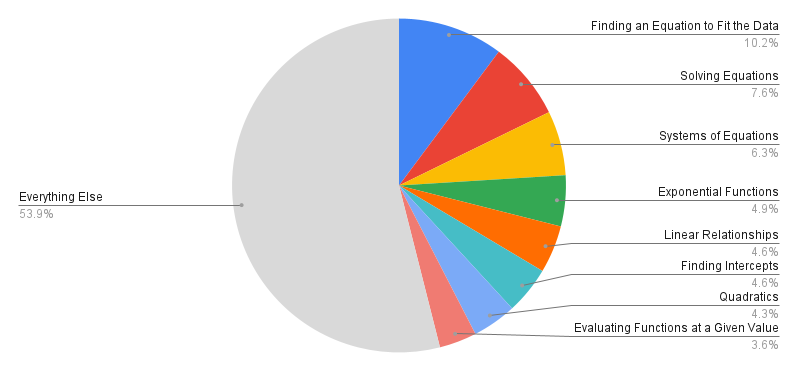Of course, this isn’t to say that you shouldn’t consider any other concepts, but rather that honing your skills on these eight topics will help you ace close to half of the math section. Since you’re probably curious, some of the concepts that appear least frequently in the new practice sections include Arc Length and Circumference of a Circle, Adding and Subtracting Fractions, and almost all things related to statistics (Range, Mean, Median, Mode, Standard Deviation, Surveys, etc.), along with a few others. You will see these concepts appear, but they’ll make up a relatively small percentage of the test.

What do these most common topics look like on the digital SAT? Here, we’ll focus on four: Finding an Equation to Fit the Data, Exponential Functions, Finding Intercepts, and Quadratics. We’ll highlight the other four in Part 2 of this series.

Finding an Equation to Fit the Data

This isn’t an official SAT term describing this first topic, but we think it pretty much sums up what each question is about: here are a few (x, y) coordinate pairs; tell us which equation fits (works with) these numbers. Here’s what it might look like: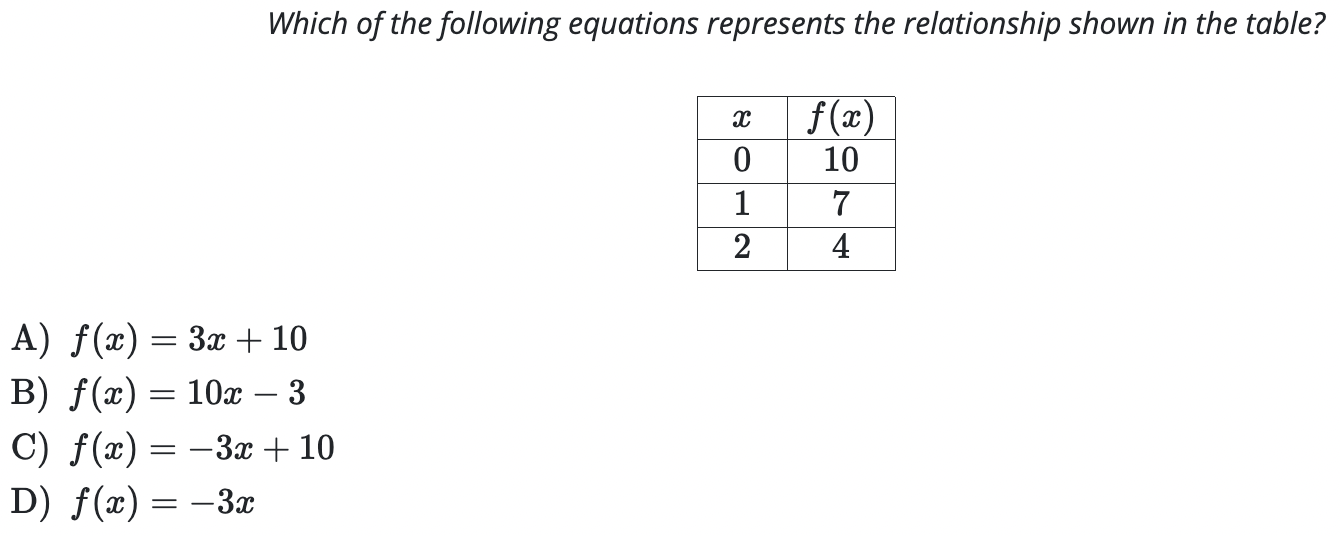As with most questions, there are several ways to get to the answer for this one, and if you’ve read our blog post on Desmos, you might recognize that this one can be solved using the graphing calculator. Instead, let’s talk about how to find the answer algebraically. Here’s the key to solving these types of problems: we can plug the three x-values given in the table into the answers. If an equation “works” for each x-value in the table, as the correct answer will, it means that plugging in all three x-values from the table into that equation will produce the corresponding  f(x)-values that the table says it should.

So if we plug x = 0 into the correct equation, the result must be 10. But since 0 times anything is 0, each time we plug in x = 0, the term with x in it will just disappear: the 3x in A, the 10x in B, and the –3x in both C and D all become 0, leaving us with just the constant term at the end of each equation. So A and C both give us 10, B gives us –3, and D gives us 0. Since we needed to get 10 after plugging in x = 0, B and D must both be wrong, so we can cross them out and are now just left with A and C.

Now we can plug in a different x from the table to find our answer. Let’s try x = 1. In A, this gives us 3(1) + 10, which equals 13, not 7 like the table says it should, so A must be wrong. To show that C is correct, we’ll also plug 1 in there: –3(1) + 10 is –3 + 10, which does equal 7. So C is our answer.

Notice that we didn’t end up testing the final x-value of 2 since we already found our answer without it; sometimes we won’t need to plug in all the x-values to get our answer, but plugging 2 into the equation in C would give us 4 (like the table says it should) if we tried it.

What’s a faster way to do this one? As the x-values increase by 1, notice that the f(x)-values in the table decrease by 3. This means that the slope of the line is –3. Since the slope in each of the answers is the number in front of x, the answer has to be either C or D. And since the y-intercept is always the point with an x-value of 0 (more on this later), the y-intercept must be 10: this is the point (0, 10) from our table. This y-intercept is the constant term at the end of each of our equations, so the equation must be f(x) = –3x + 10, giving us our answer: C. This way is much faster if you know what you’re looking for!

Exponential Functions

Linear functions increase or decrease at a constant rate, whereas exponential functions do so at a rate that’s not constant, but rather a percentage change. Populations grow exponentially, as does the value of money growing with interest. The equation y=50(1.08)t is an example of an exponential function; the giveaway is the variable, t, in the exponent. In these functions, t usually represents time, which is often measured in years.

Now let’s analyze this function. The number out in front, 50, is the initial value of the function. So when t = 0, at the very beginning when no time has passed, the function starts at a value of 50. If this function represented a population of people, for instance, the initial size of the population would be 50.

The 1.08 in the parentheses represents how quickly the function is growing. 1.08 is the same thing as 1.00 + 0.08, or 100% + 8%, so this function is growing at a rate of 8% per year (if the number in the parentheses were less than 1, like 0.70, for example, the function would be decreasing; in this case, it would decrease 30% per year: 0.70 is 1.00 – 0.30, or 100% – 30%).

Here’s an example of how this topic might show up on the SAT.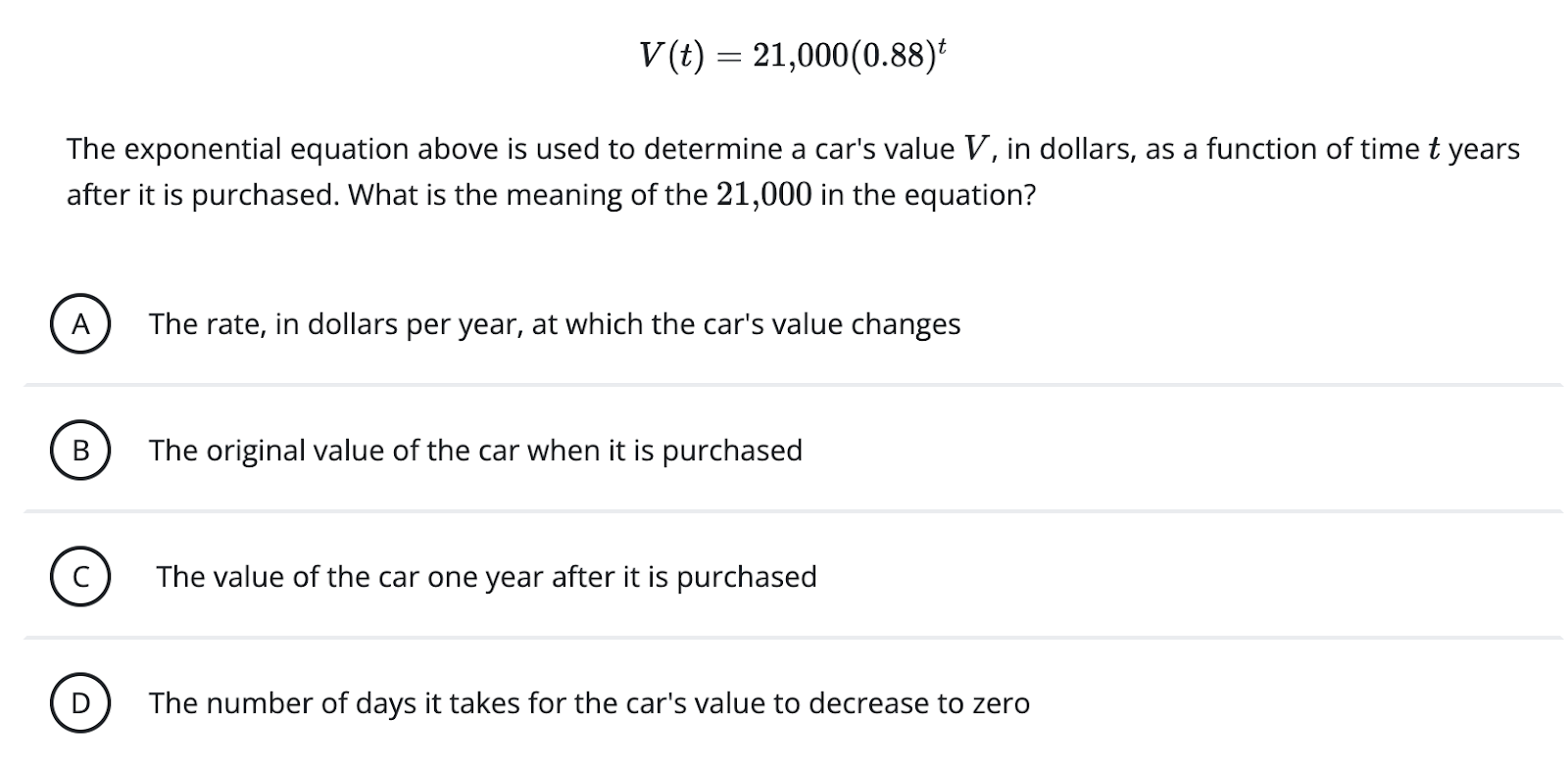This one should be pretty straightforward, especially after what we just talked about; the number out front, 21,000, is always the initial value of the function, so here, since we’re talking about the value of a car, the car must have been worth \$21,000 when it was first purchased. And that corresponds to answer B.

They could have asked us about the meaning of the 0.88 in parentheses, in which case we’d say that 0.88 is the same as 1.00 – 0.12, or 100% – 12%, so in this context, that means the car decreases in value by 12% each year.

Finding Intercepts

Questions that fall under this topic can often be solved using Desmos, as can most questions from topics dealing with graphs, but here we’ll use algebra to cover the basics.

A graph has an x-intercept where it crosses the x-axis and a y-intercept where it crosses the y-axis. How do we find these intercepts? Each point on the x-axis has a y-value of 0. For example, the points (5, 0), (12, 0), and (–100, 0) are all on the x-axis. This means that plugging y = 0 into a function will always give us a point on the x-axis, which means that point is the x-intercept of that function. Similarly, plugging x = 0 into a function always gives us the y-intercept, since all points on the y-axis have x-values of 0.

Let’s apply this strategy to an SAT question.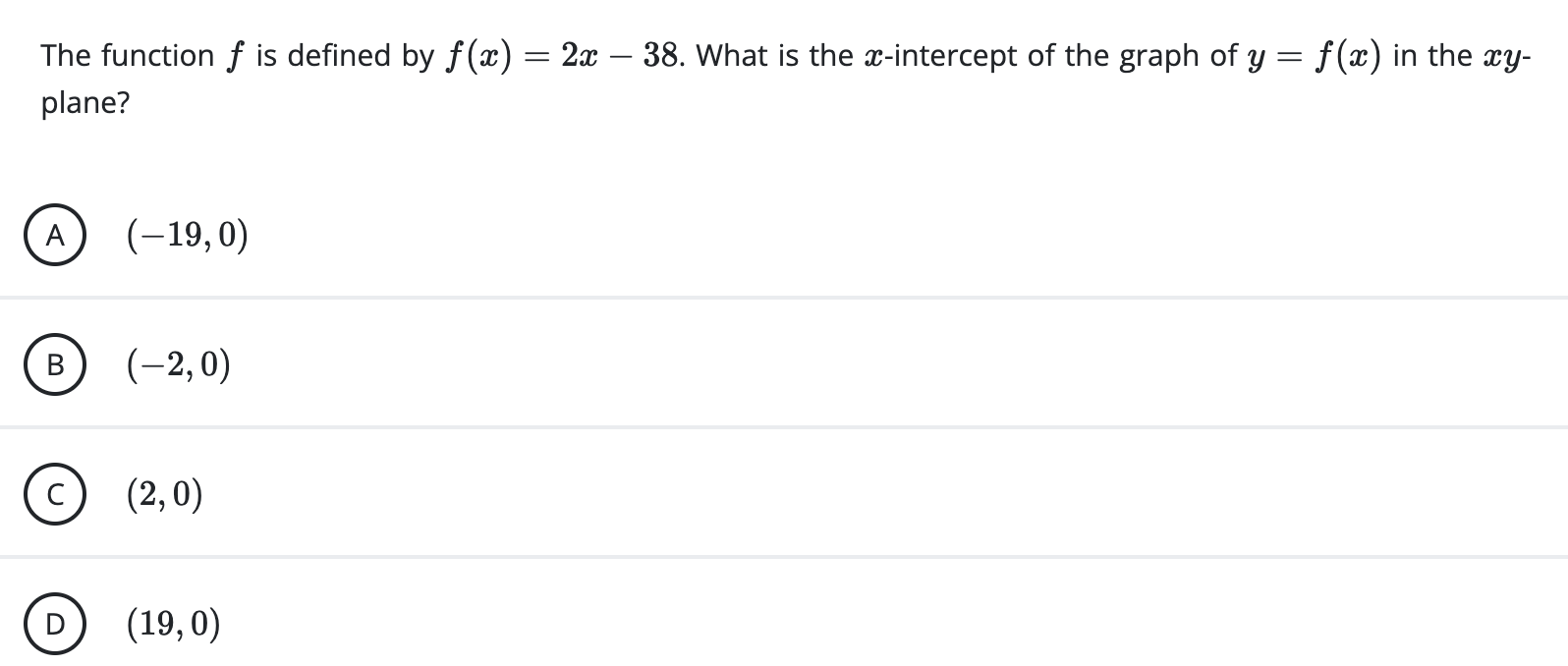As we mentioned earlier, we could definitely put this equation into Desmos and find its x-intercept, but we could also do what we just mentioned and plug in y = 0 to find the x-intercept. Anytime we see f(x), we could replace it with y because y and f(x) are interchangeable. Since we want to plug in y = 0, we can therefore replace f(x) with 0 to do the same thing:A quadratic equation has x2 as its highest-power term. For example, y = x2 + 5x + 6 is a quadratic equation. The graph of a quadratic is called a parabola, a curve that either opens upward (in the shape of a U) or downward. The number of times the parabola crosses the x-axis is the number of solutions to the quadratic equation when y = 0; there can either be zero, one, or two solutions for a quadratic. The SAT loves questions involving this concept. Here’s one.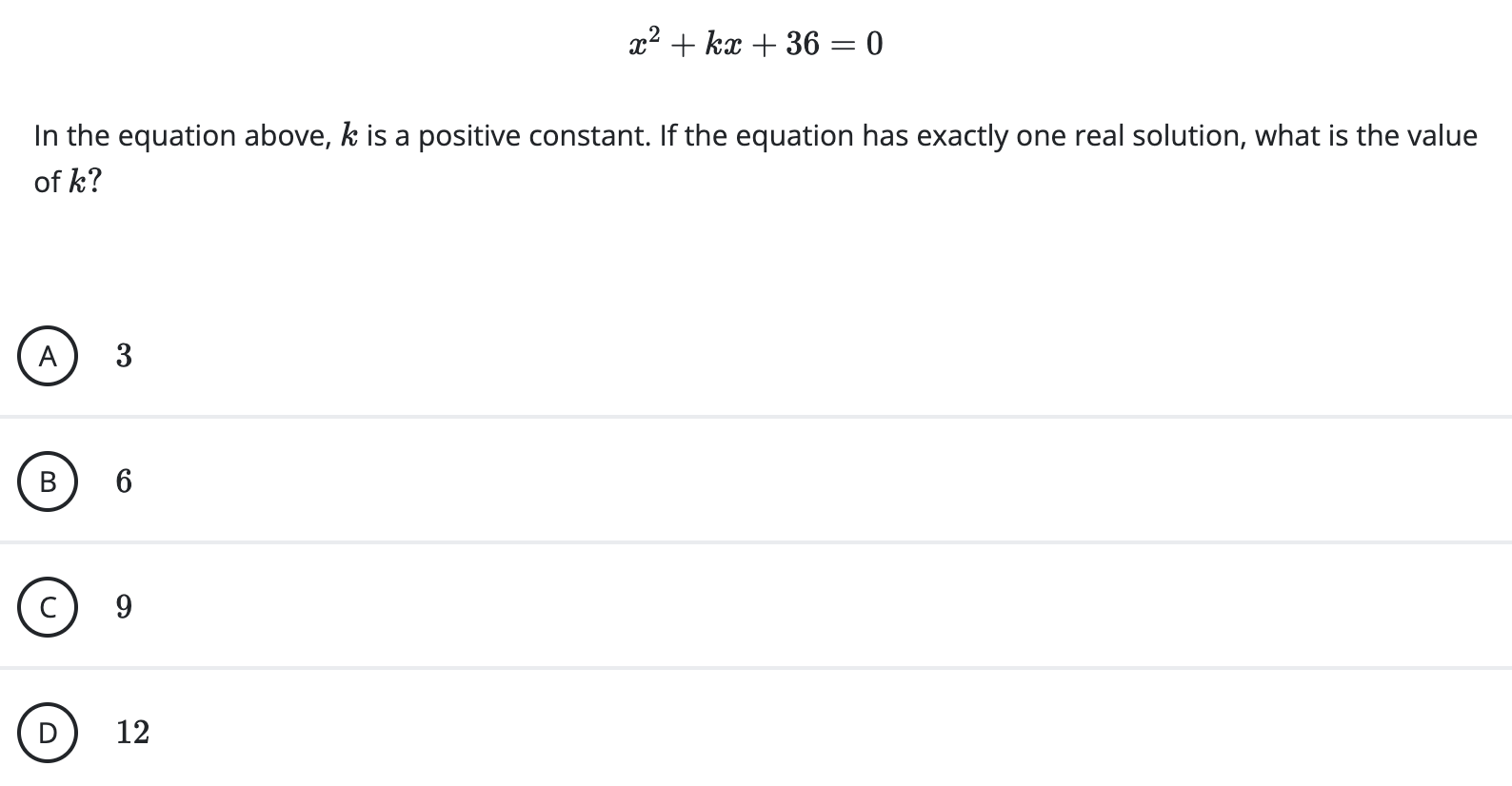Before we tackle this one, let’s talk about the quadratic formula, which looks like this:(where a, b, and c are the three coefficients of the quadratic equation). The part inside of the radical, b2 – 4ac, is called the discriminant. If the discriminant is positive, the quadratic has two solutions (two x-intercepts). If the discriminant equals zero, we’ll get one solution. And if the discriminant is negative, the quadratic equation has no solution.

In our question, notice we’re told that “the equation has exactly one real solution.” This is code for the discriminant equals zero! In fact, any time they mention a number of solutions in a quadratic question, we can use the discriminant. So we can set our discriminant equal to zero and solve the resulting equation to find the value of k. From our quadratic, we know that a = 1, b = k, and c = 36: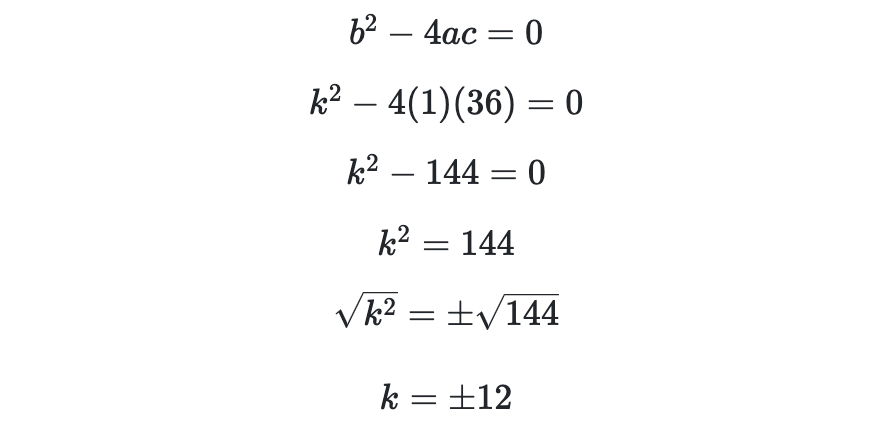Since the problem tells us that k is positive, it must be 12, so our answer is D. There are other variations to the quadratic problems that we’ll see on the SAT, but this one involving the discriminant shows up pretty often.